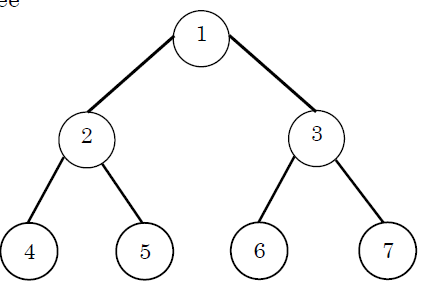Skip to content
Related Articles
ISRO | ISRO CS 2017 – May | Question 49
• Last Updated : 21 Mar, 2018

Consider the following treeIf the post order traversal gives ab-cd*+ then the label of the nodes 1,2,3,… will be
(A) +,-,*,a,b,c,d
(B) a,-,b,+,c,*,d
(C) a,b,c,d,-,*,+
(D) -,a,b,+,*,c,d

Answer: (A)

Explanation: Postorder traversal of the given binary tree will give the following sequence: 4 5 2 6 7 3 1.
Now comparing the sequence with a b – c d * + we get 1 = +, 2 = -, 3 = *, 4 = a, 5 = b, 6 = c and 7 = d.
So, option (A) is correct.

Quiz of this Question

My Personal Notes arrow_drop_up